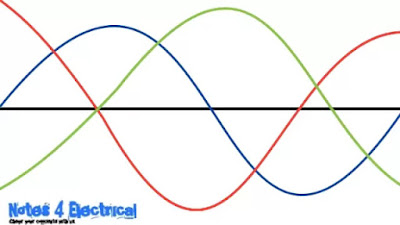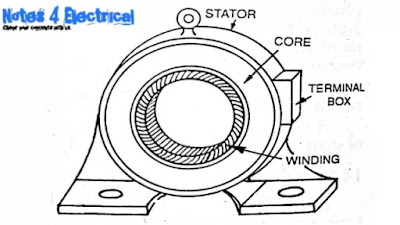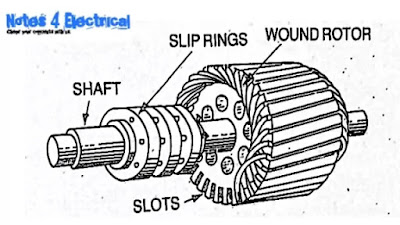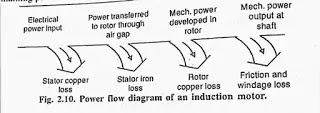# Induction motor, Types of induction motor, How induction motor works

Hello guys, hope you are all good and well. Today we will discuss about,

• What is induction motor
• Types of induction motor
• Construction of induction motor
• How induction motor works?
• Power flow diagram of induction motor.

So let's start,

## What is the meaning of induction motor?

As there are mainly two words used first is induction and other is a motor so what is the meaning of both these words?

Let’s understand the meaning of induction as you know that this motor works on the principle of electromagnetic induction therefore the word induction is used.

And the motor is the electrical machine which converts electrical energy into machanical energy.

So that’s why it is called induction motor. The other name of this motor is asynchronous motor.

So again a question arises here that why it is called asynchronous motor?

The motor does not run at synchronous speed therefore, it is called asynchronous motor.

## What is the synchronous speed?

The speed of the in the induction motor is called synchronous speed. It is generally denoted by Ns. And given by,

Ns=120f/p

Where,
Ns= synchronous speed in rpm
f= supply frequency in Hz
p= no. of poles

So do you know why there is 120 is taken? Why other is quantity is not taken..
So want to know about it???

So here is your answer there is 120 is taken because the phase angle between the conductors is 120° (in three phase system).Fig. Angle between the phases

## What is an induction motor : -

This is the most common type of Ac motor. It converts AC electrical power into mechanical power. As discussed earlier, it is also known as asynchronous motor. These motors are more rugged, require less maintaincace, less expensive, and high efficiency.

## TYPES OF INDUCTION MOTOR: -

There are two types as given below:-

• Single phase IM – not self starting.
• Three phase IM – self starting (three phase induction motor are widely used for industrial applications).

## CONSTRUCTION OF INDUCTION MOTOR: -

There are mainly two parts: -
• Stator
• Rotor

### Stator: -

The Stator is the  stationary part of the motor and it has mainly three parts as given below –Add caption

Stator frame: - the frame is used to support the core and the windings. It may be cast or fabricated steel. For small and medium size machines, it’s cast and for large machines it is fabricated.

Stator core: - the core is the place where winding is placed or accommodate. The slots are provided to place them and it is made up of laminations.

Stator winding: - three phase winding are placed in the stator core slots. The winding may be star connected or delta connected.

### ROTOR: -

It is the rotating part of the motor. And it consist mainly two parts: -Fig. Rotor of induction motor

Rotor core: - It is made up of laminations and placed on the shaft. The slots are made on the core, but these slots are not cut in the axial directions (slots are made skewed) because of the following reason: -
1. To reduce noise
2. To reduce Stalling of motor (in Hindi= मोटर का अटकना)
3. Tendency of locking of rotor is reduced.
Now what’s the meaning of point no. 3 i.e. tendency of locking of rotor is reduced??(We will discuss about it later).

Rotor conductor: - the rotor conductors or windings are may be bars or slip ring winding.

Now let us talk about the types of rotor used in induction motors: -

There are mainly two types used–
1. Squirrel cage rotor
2. Slip ring cage rotor

## WORKING PRINCIPLE (for three phase IM ): -

Let understand this in some steps: -

1. When three phase supply is given to the stator an current flow through the stator winding which produce rotating magnetic field.

2. This rotating magnetic field links with the rotor conductor and according to the electromagnetic induction an emf is induced in the rotor bars or conductors and as we know that the bars are short circuited by the end rings so an current flow through the rotor.

3. This rotor current produces its own magnetic field which opposes the main magnetic field (according to L).

4. Hence we know that when a current carrying conductor (means rotor) is placed in the varying magnetic field (rotating magnetic field) a torque is produced in it (in the rotor). And the it starts to rotate in the direction of rotating magnetic field.

Now, as we discussed above about the locking. Are you excited to know about it?

## What is locking of the rotor?

As we have discussed in the working that When the supply is given to the induction motor then a rotating magnetic field produced.

Due to this rotating magnetic field an emf is also produced in the rotor due to which a current will starts to flow in the it.

And a magnetic field is also produced in the rotor due to this current. When this rotor magnetic field comes into the locking with the rotating magnetic field of the stator then It is called locking of the rotor.

The locking is the undesirable situation if rotor locks, there is no emf induced in it, because we know that emf will induce only when the rotating magnetic field cuts the rotor.

The emf will not induced in the rotor also there is no current in the rotor due to which it will not rotate.

Well rotor doesn’t interlock with the stator field in real practice.

So why it is not interlocked???

Ans. Due to the lag (means rotor follows the stator) between the flux current in the rotor and the flux current in the stator, the rotor will never reach its rotating magnetic field speed (i.e. the synchronous speed).

Now, as we know that the frequency of the stator is the supply frequency means let assume supply frequency  is 50 Hz then stator frequency will also be 50Hz. But what will the frequency of the rotor??
So there is an formula by which we can find

frequency of the rotor as given below:-

F'=SF

Where,

F'= frequency of the rotor
S= slip
F= supply frequency

So will will discuss about the slip and significant of slip in the next article.

## Power flow diagram of the induction motor:-

It is the diagram in which we can clearly see that how the electrical power supplied to the stator winding is converted into mechanical power. Some of the part of this electrical power is lost in copper loss and iron loss (in the form of heat).Fig. Power flow diagram of induction motor

Copper loss= 3.5%
Iron loss=2.5%

And the remaining power,  i.e. 94% is converted into the machanical power. And then windage losses and friction losses occur at the output (shaft). These two losses are negligible.

Wanna learn about single phase induction motor, it's types, construction and working.

Wanna learn about three phase induction motor, slip, significance of slip and reversal of rotation of three phase induction motor.

If you like the article then share with your friends.

Thank you

Hope the article helps you.